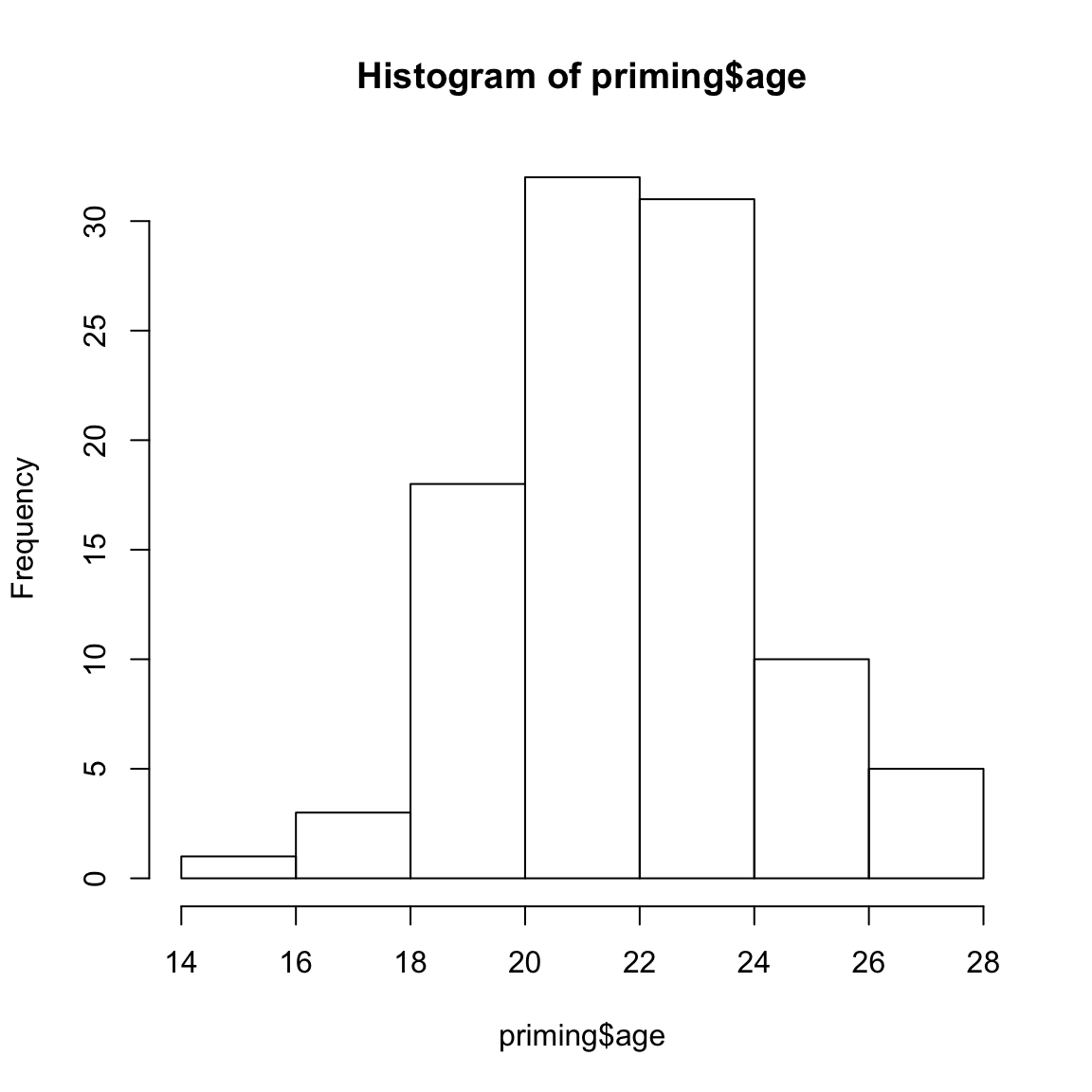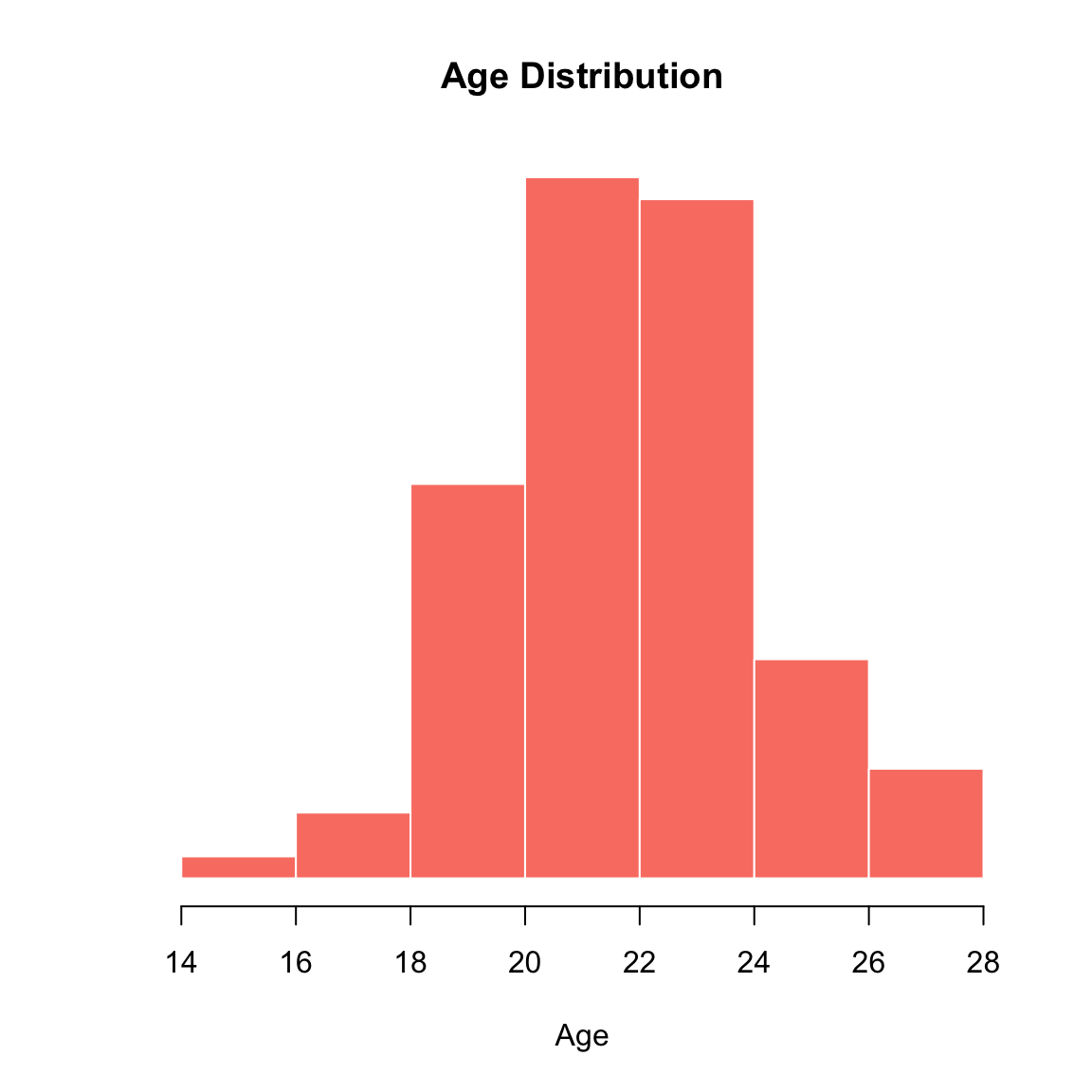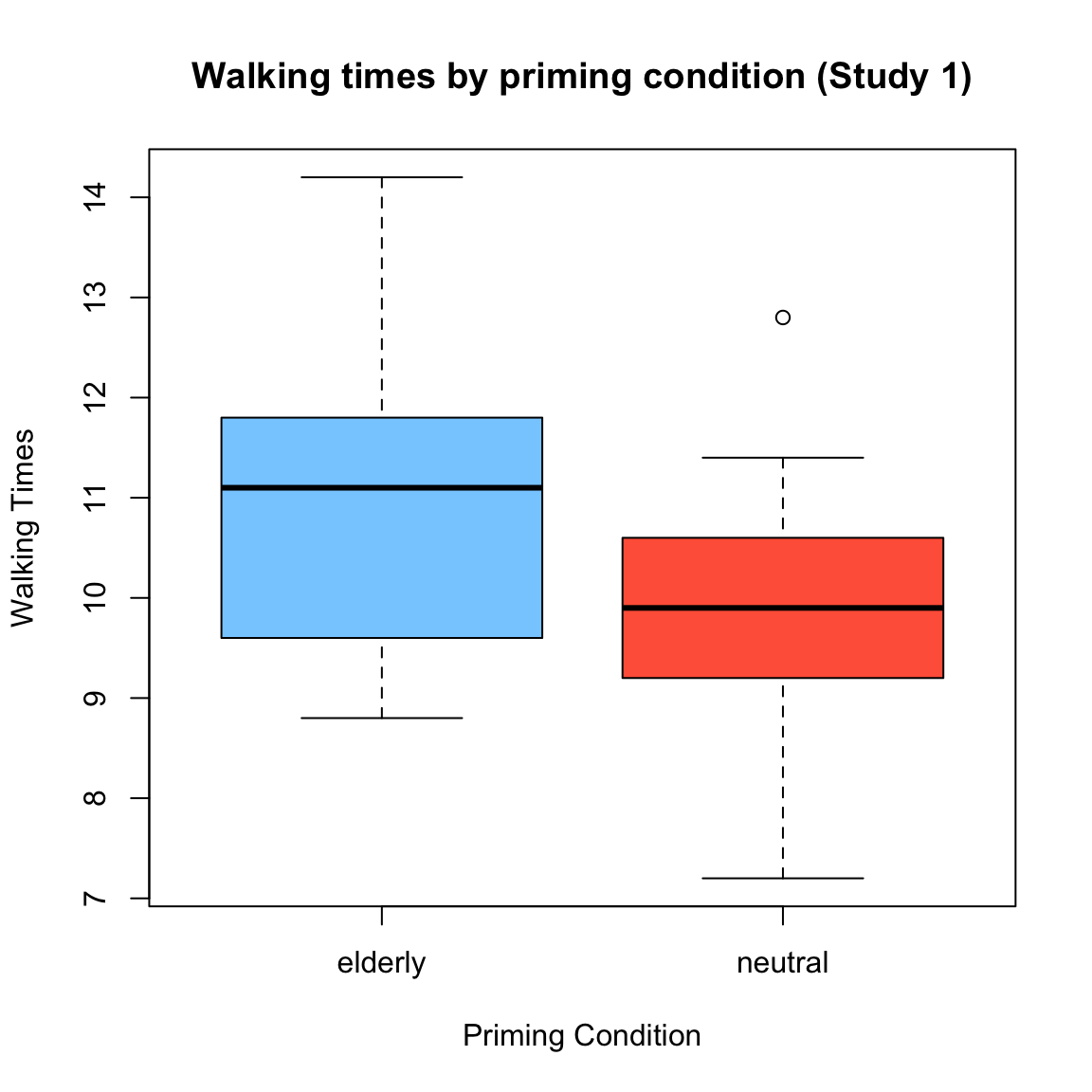# 0: Install / Update R and RStudio

Before you do anything else, make sure you’ve got the latest versions of R and RStudio installed!

# 0.5 Update Language

If you want to change the laguage in RStudio to English, run the following code:

``Sys.setenv(LANG = "en")``

# 1: Create and view data objects

Everything in R is an object with a name. Everything you do in R is a function. Let’s create a new object called my.data some experimental data. The data is stored as a text file at the web link https://dl.dropboxusercontent.com/u/7618380/priming.txt

``````priming <- read.table(file = "https://dl.dropboxusercontent.com/u/7618380/priming.txt",
sep = "\t",   # File is tab-delimited
)``````

You can look at the data using the View() function applied to the priming object

``````# Show the priming dataset in a 'spreadsheet'
View(priming)``````

You can get summary information about the data using the summary() function

``````# Calculate summary statistics of each column
summary(priming)``````
``````       id         sex         age            prime         time
Min.   :  1.00   f:53   Min.   :14.00   elderly:50   Min.   : 7.200
1st Qu.: 25.75   m:47   1st Qu.:21.00   neutral:50   1st Qu.: 9.375
Median : 50.50          Median :22.00                Median :10.300
Mean   : 50.50          Mean   :22.21                Mean   :10.337
3rd Qu.: 75.25          3rd Qu.:23.00                3rd Qu.:11.225
Max.   :100.00          Max.   :27.00                Max.   :14.200  ``````

# 2: Calculate summary statistics

You can calculate simple statistics using functions like mean(), sd(), and table()

``````# Calculate some basic summary statistics of specific columns

mean(priming\$age)   # What was the mean age of the participants?``````
`` 22.21``
``median(priming\$time)  # What was the median time?``
`` 10.3``
``table(priming\$sex) # How many people were there of each sex?``
``````
f  m
53 47 ``````

# 3: Plotting

Plotting is super easy in R!

``````# Simple histogram of age
hist(x = priming\$age)``````You can customize the look of your plot with additional plotting arguments

``````# Age histogram with additional arguments

hist(x = priming\$age,
border = "white",
col = "salmon",
main = "Age Distribution",
xlab = "Age",
yaxt = "n",
ylab = ""
)``````Here’s a boxplot:

``````# Boxplot of times by priming condition

boxplot(formula = time ~ prime,
data = priming,
col = c("skyblue1", "tomato"),
ylab = "Walking Times",
xlab = "Priming Condition",
main = "Walking times by priming condition (Study 1)"
)``````Now a scatterplot!

``````# Scatterplot of age and time

plot(x = priming\$age,
y = priming\$time,
pch = 16,             # point type
col = gray(.5, .5),   # point color
cex = 2,              # point sizes
bty = "n",            # remove outer box
xlab = "Age",
ylab = "Time",
main = "Age and Walking times\nStudy 1"
)